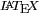This site is a showcase for using LatexRender for mathematics in WordPress

# Using LaTeX in WordPress

## Thursday 21st July 2005

Filed under: — Steve @ 10:33 am

WordPress is very flexible allowing you to choose various text filters. Unfortunately they can filter the mathematical text as well before it gets converted to an image. The most common effect you see is in matrices (or arrays, tables) where you get

This is because the ampersand has been converted to the equivalent HTML code.

You can solve this problem by changing one line in latex.php. In
// $latex_formula = str_replace("& #38;","&",$latex_formula);
remove the comment // and change 38 to 038 so that the line reads
$latex_formula = str_replace("& #038;","&",$latex_formula);
Please note: In order to show the code here I have had to introduce a space between & and #. That space should not be there in latex.php

Other odd effects can be dealt with similarly.
If you see a8217; instead of a’ then either
1. use ^\prime in your LaTeX code and/or
2. add the line (removing the space between & and #)
$latex_formula = str_replace("& #8217;","'",$latex_formula);
in the same place as the previous one in latex.php

Note: If you want to regenerate older images then you will either have to remove them from the cache or change the code slightly eg by putting in an extra space whichwill ignore

1. I just installed latexrender, using mimetex, on WP 2.1.3, and ran into a couple of “odd effects”: ampersands in formulae were being treated as “& amp” (without the space), multiple spaces were being treated as capital-A-circumflex, and spaces were being treated as “& nbsp” (without the space).

To resolve this, I read the above post and added the following three lines after line 29 in mimetex.php to undo WP’s:
 $mimetex_formula = str_replace("& amp;","&",$mimetex_formula); $mimetex_formula = str_replace("& nbsp;"," ",$mimetex_formula); $mimetex_formula = str_replace("Ã‚","",$mimetex_formula); 
(remove the spaces before “amp” and “nbsp”; I had to add spaces for this comment to display correctly)

Great program once I got it working! Hope this comment will be helpful for others.

Comment by TG — Sunday 29th April 2007 6:45 pm #

2. I think I’ve found a simpler solution to conflicting plugins. I ran into similar artefacts when using the Markdown Extra plugin with latexrender and figured that it was due to Markdown converting ampersands into “amp” entities before latexrender could extract equations from the text.

The solution was to change the priority of latex render to something higher than that of markdown. The WordPress Plug-in API says you can do this with the optional priority argument for the add_filter command. Specifically, I changed lines 18-20 of latexrender-plugin.php to
 add_filter('the_title', 'addlatex', 5); add_filter('the_content', 'addlatex', 5); add_filter('the_excerpt', 'addlatex', 5); 

The number “5” was chosen arbitrarily. According to the API docs anything less than 10 (the default plug-in priority) would have worked.

Comment by Mark — Monday 4th February 2008 10:48 pm #

3. I’m having a lot of trouble with LaTeX matrices in wordpress.com – do you know if something similar can be made to work here as well?

Comment by amca — Monday 19th May 2008 12:05 am #

4. Sorry about the delay in replying. I have seen your question at http://en.forums.wordpress.com/topic.php?id=28520. Instead of using array it is simpler to use the pmatrix environment and it appears that it works much better on wordpress.com.

So replace
$latex \left(\begin{array}{c}x'\\ y'\\ z'\\ w'\end{array}\right)=\left(\begin{array}{cccc}1&0&0&0\\ 0&1&0&0\\ 0&0&\cos\theta&-\sin\theta\\ 0&0&\sin\theta&\cos\theta\end{array}\right)\left(\begin{array}{c}x\\ y\\ z\\ w\end{array}\right)$
by
$latex \begin{pmatrix}x'\\ y'\\ z'\\ w'\end{pmatrix}=\begin{pmatrix}1&0&0&0\\ 0&1&0&0\\ 0&0&\cos\theta&-\sin\theta\\ 0&0&\sin\theta&\cos\theta\end{pmatrix}\begin{pmatrix}x\\ y\\ z\\ w\end{pmatrix}$

Note the sace before the first 0 on the fourth row of the second matrix to prevent wordpress.com misreading it.

Comment by Steve — Thursday 22nd May 2008 11:17 am #